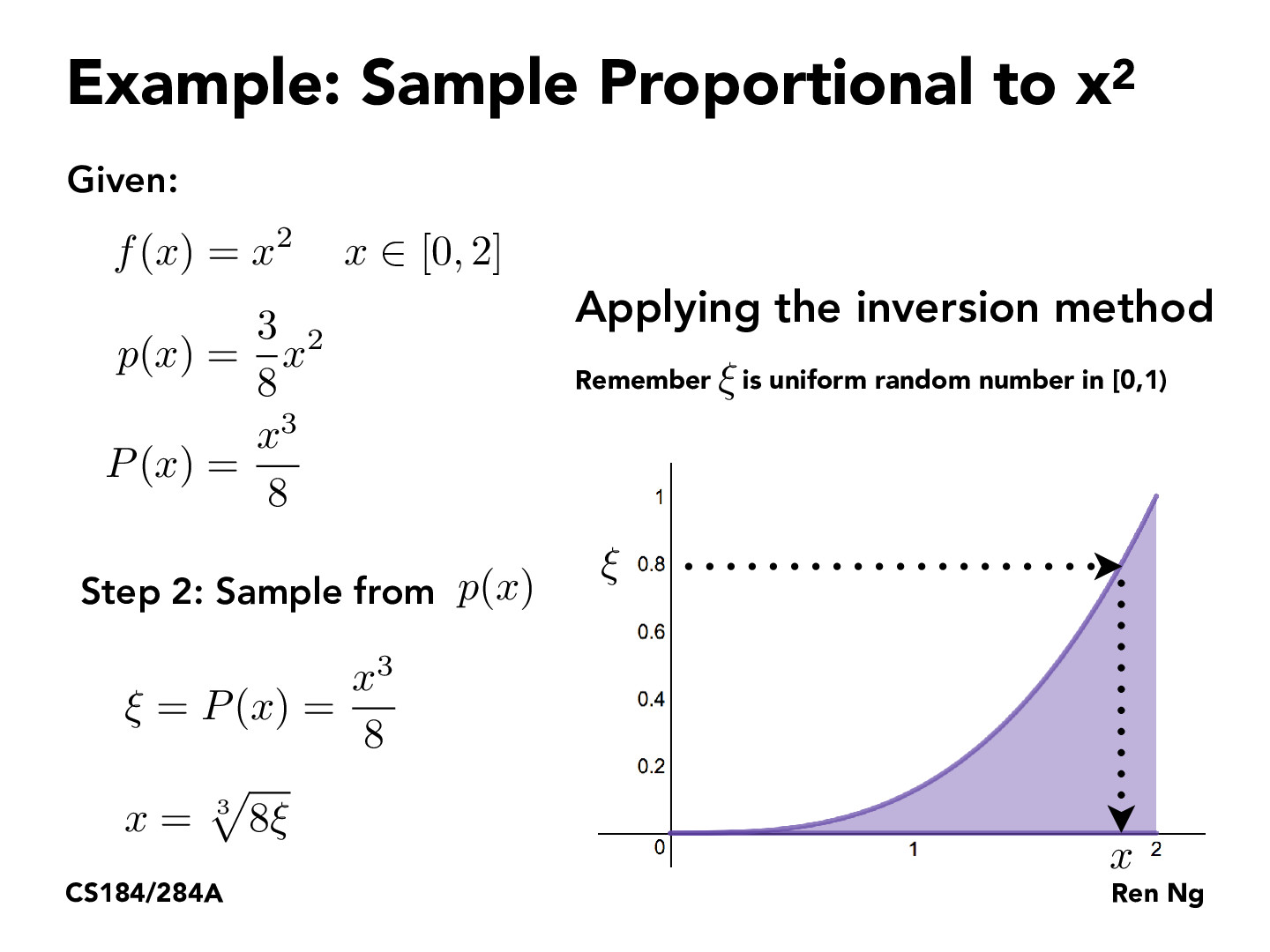Lecture 12: Monte Carlo Integration (55)jcebreros1

This makes sense and all but isn't there already libraries that will gives us an RV in a desired distribution? For example, in python you can use numpy.random.desired probabilitydistribitionet-yao

That's probably how they work on the inside, which is always very nice to know.stecen

I was having trouble grasping the intuition on how this step 2 here would generate samples according to the original PDF distribution, because the CDF encodes the "likelihood" to sample all numbers <= x, rather than the likelihood of actually sampling the number x. So in my incorrect mental model, if this method were to generate many samples of x, it wouldn't be because x itself has high "mass" in the pdf, but because all numbers <= x have high "mass" in the pdf. But now I've worked out an example for a uniform pdf on paper and am trying to convince myself that this intuition extends to all pdfs.

You must be enrolled in the course to comment